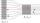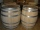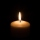# Velocity + cylinder - math problems

#### Number of problems found: 7

• Velocity ratioDetermine the ratio at which the fluid velocity in different parts of the pipeline (one part has a diameter of 5 cm and the other has a diameter of 3 cm), when you know that at every point of the liquid is the product of the area of tube [S] and the fluid
• Water flowHow much water flow in pipe with a diameter of 16 cm in 1 hour if the water velocity is 2.5 m/s?
• Two cylindersTwo cylinders are there one with oil and one with an empty oil cylinder has no fixed value assume infinitely. We are pumping out the oil into an empty cylinder having radius =1 cm height=3 cm rate of pumping oil is 9 cubic centimeters per sec and we are p
• Water flow 2How many litres of water will flow in 7 minutes from a cylindrical pipe 1 cm in diameter, if the water flows at a speed of 30 km per hour
• Water tankThe water tank has a cylindrical shape with a base diameter of 4.2 m and is 80 cm deep. How many minutes will take fill it 10 cm below the edge of the tank if water flowing 2 liters per second?
• PipesThe water pipe has a cross-section 1087 cm2. An hour has passed 960 m3 of water. How much water flows through the pipe with cross-section 300 cm2 per 9 hours if water flows the same speed?
• CandlesBefore Christmas, Eva bought two cylindrical candles - red and green. Red was 1 cm longer than green. She lit a red candle on Christmas Day at 5:30 p. M. , lit a green candle at 7:00 p. M. , and left them both on fire until they burned. At 9:30 p. M. , bo

We apologize, but in this category are not a lot of examples.
Do you have an interesting mathematical word problem that you can't solve it? Submit a math problem, and we can try to solve it.

We will send a solution to your e-mail address. Solved examples are also published here. Please enter the e-mail correctly and check whether you don't have a full mailbox.

Please do not submit problems from current active competitions such as Mathematical Olympiad, correspondence seminars etc...

Do you want to convert velocity (speed) units? Velocity - math word problems. Cylinder Problems.